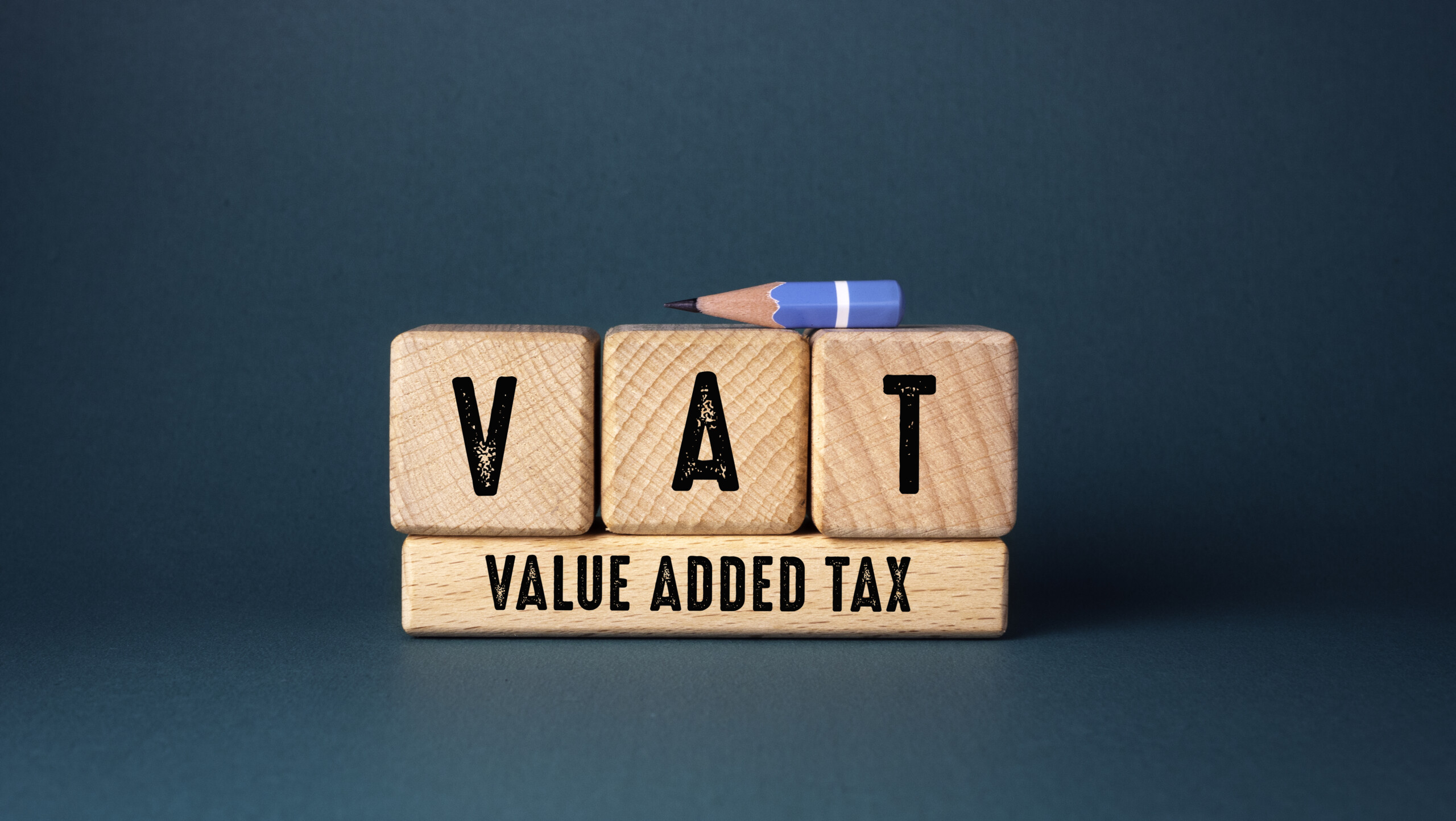Worried about how much VAT to add? Received a price including VAT but don’t know exactly how much VAT?

Online VAT Calculator Helps you calculate VAT excluding VAT and including VAT. Just enter the value and we’ll calculate the VAT for you.
How the
VAT Calculator Works You can use the
VAT Calculator to determine your taxable and VAT amounts. To calculate VAT, you only need to know the amount, i.e. the price, and the VAT rate. The VAT Calculator allows you to calculate exclusive and inclusive VAT.

Excluding VAT
The amount or price to enter, excluding VAT, is displayed here. H. It is purely the cost of the product or service. If you select “Exclude VAT”, the VAT rate will be applied according to the formula below.

Taxable amount = VAT rate ÷ 100

VAT included
The amount or price stated here includes VAT. That is, it includes the product price and the value added tax amount. If the displayed price includes VAT, the VAT amount can be determined using the following formula:

VAT amount = tax-inclusive price)

What is input tax and input tax?
The basis of VAT is mainly based on the concept of input tax and output tax. Let’s understand with an example.

Sales Tax

A tax levied on the sale of goods or services is known as sales tax. A-One Traders, for instance, sold items for \$1,000,000 plus 5% tax. A-One Traders collected a tax of \$5,000 in an output vat.

Input VAT

Input tax is the term for the tax paid at the time goods or services are purchased. For instance, Jumbo Distributors sold items to A-One Traders for \$50,000 plus 5% tax. A-One Traders’ 2,500 tax payment is an example of an input tax.

How to calculate VAT payment?
VAT Registered businesses must pay VAT, but this does not mean they have to pay the full amount of VAT collected on their sales. Below is a formula that you can use to determine or calculate the VAT you pay to the state.

Output VAT – (Minus) = VAT Payment Enter VAT

All you need to do now is calculate the total output VAT collected during the tax period and the total input VAT that can be recovered. After calculating the output sales tax and input sales tax, apply the above formula.

If the output VAT is higher than the input VAT, the difference is the amount of VAT paid. If the output VAT is lower than the input VAT, the amount will be refunded and no VAT will be charged.

You can also register for VAT Registration on our website:
https://www.vat-registration-uae.com/